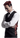# Red Ochsenbein

## React: Smoothly move the seconds hand of the analog clock

In my last article about the analog clock I showed how I built it. The seconds hand is just jumping from second to second but I wanted to have it move smoothly.

The code for the seconds hand:

``````const Seconds = styled(Hours).attrs<DateProps>(({ time }) => ({
style: { transform: `rotateZ(\${time.getSeconds() * 6}deg)` },
}))<DateProps>`
background-color: red;
height: 50px;
left: calc(50% - 0.5px);
top: 5px;
width: 1px;
```````

## First try

My immediate thought was to simply add `transition: transform 1s linear;` to the css.

``````const Seconds = styled(Hours).attrs<DateProps>(({ time }) => ({
style: { transform: `rotateZ(\${time.getSeconds() * 6}deg)` },
}))<DateProps>`
background-color: red;
height: 50px;
left: calc(50% - 0.5px);
top: 5px;
transition: transform 1s linear;
width: 1px;
```````

That was it! Job done…

Unfortunately not.

At first it looked good until the hand moved from 59 seconds to 0. What happens with the calulation?

``````59 * 6 = 354
0 * 6 = 0``````

This means the hand does not move from 354 degrees to 360 degrees, but to 0 degrees. This means it runs backwards. One full rotation back to zero in 1 second. While this might be somewhat funny it is not what I wanted (or anyone would expect from a clock).

Also there is another small problem with this: The hand is one second behind. Think about it: When we jump from position to position the hand is at the target position (the position of the current second) immediately. When transitioning within a second it starts at the previous second and takes a second to get to the current position. Anyways this could have been easily fixed by just adding 1 to `time.getSeconds()`.

But we can do better.

After jumping to the first solution that came to mind I started thinking about other possible ways to do this.

What if I just did not use `time.getSeconds()` but just `Date.now()`? It should get me the milliseconds since January 1st 1970. Dividing it by 1000 and using that should do the trick.

Unfortunately it’s not that easy. The generated div looked like this:

``<div class="sc-cxabCf bIucpB" style="transform: rotateZ(9.96329e+09deg);"></div>``

The number was simply too big. Also I’m not sure if using something like 9963290976 degrees to start with might be a good practice.

Other solutions I was thinking of were:

• Add a modulo of 86400 which would reset the value to 0 every 24 hours. Then the reversing of the hand would only happen once a day at midnight. Better, but I did not want any reversing at all.
• Storing the starting moment and calculating the difference between now and the start (`Date.now() - start`). This solutions seems reasonable so I went on and tried it.

## Using the time delta

To calculate the difference bettween the start time and now I had to store the starting point somewhere. Since this should not change at all and it should not retrigger a rerender I am using a ref for this.

``````const DemoClock: React.FC = () => {
const [time, setTime] = useState(() => new Date())
const start = useRef(time)

useEffect(() => {
const interval = setInterval(() => {
const now = new Date()
if (time.getSeconds() !== now.getSeconds()) {
setTime(now)
}
}, 250)

return () => clearInterval(interval)
}, [time])

const seconds = Math.floor((time.getTime() - start.current.getTime()) / 1000)
+ start.current.getSeconds() + 1

return (
<Clock>
<Hours time={time} />
<Minutes time={time} />
<Seconds seconds={seconds} />
</Clock>
)
}``````

This works just fine. But one thing still bothered me. The degrees are growing larger and larger. I don’t think this is a problem but I wanted to try a solution which does not need these large numbers.

## Using the Web Animation API

I ended up doing this:

``````const DemoClock: React.FC = () => {
const [time, setTime] = useState(() => new Date())

const secondsRef = useRef<HTMLDivElement>(null)

useEffect(() => {
const interval = setInterval(() => {
const now = new Date()
if (time.getSeconds() !== now.getSeconds()) {
setTime(now)
}
}, 250)

return () => clearInterval(interval)
}, [time])

useLayoutEffect(() => {
if (!secondsRef.current) {
return
}

secondsRef.current.animate(
[
{
transform: `rotateZ(\${(time.getSeconds() * 6}deg)`,
},
{
transform: `rotateZ(\${(time.getSeconds() + 1 * 6}deg)`,
},
],
{
duration: 1000,
fill: 'both',
iterations: 1,
}
)
}, [time])

return (
<Clock>
<Hours time={time} />
<Minutes time={time} />
<Seconds time={time}  ref={secondsRef} />
</Clock>
)
}``````

As you can see by defining the start and end of each step we never fall into the situation to have to move backwards. But also it allows us to change the time at any point without having the hand rotating like a maniac and we never need to use degrees larger than 360. To me this felt like the ideal solution.

© 2021 – 2023 Red Ochsenbein